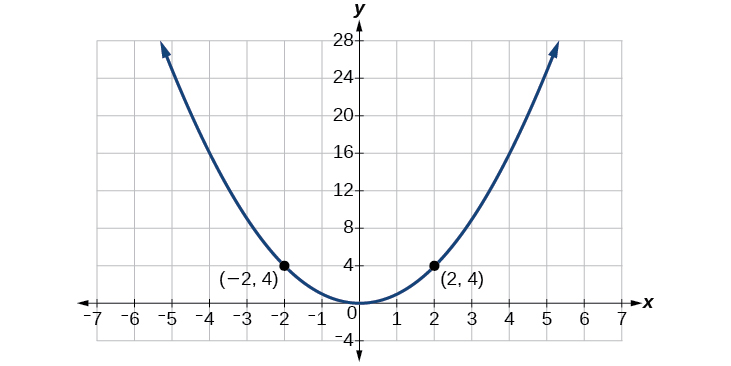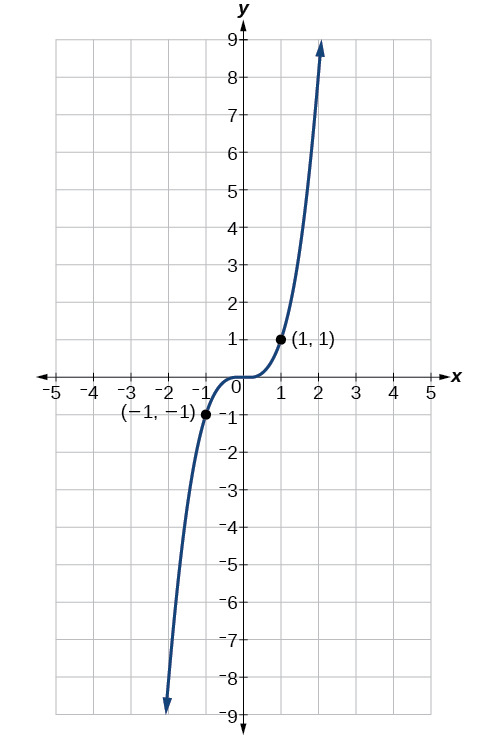# 5.3 The other trigonometric functions  (Page 3/13)

 Page 3 / 13

Use reference angles to find all six trigonometric functions of $\text{\hspace{0.17em}}-\frac{7\pi }{4}.\text{\hspace{0.17em}}$

$\mathrm{sin}\left(\frac{-7\pi }{4}\right)=\frac{\sqrt{2}}{2},\mathrm{cos}\left(\frac{-7\pi }{4}\right)=\frac{\sqrt{2}}{2},\mathrm{tan}\left(\frac{-7\pi }{4}\right)=1,$
$\mathrm{sec}\left(\frac{-7\pi }{4}\right)=\sqrt{2},\mathrm{csc}\left(\frac{-7\pi }{4}\right)=\sqrt{2},\mathrm{cot}\left(\frac{-7\pi }{4}\right)=1$

## Using even and odd trigonometric functions

To be able to use our six trigonometric functions freely with both positive and negative angle inputs, we should examine how each function treats a negative input. As it turns out, there is an important difference among the functions in this regard.

Consider the function $\text{\hspace{0.17em}}f\left(x\right)={x}^{2},$ shown in [link] . The graph of the function is symmetrical about the y -axis. All along the curve, any two points with opposite x -values have the same function value. This matches the result of calculation: $\text{\hspace{0.17em}}{\left(4\right)}^{2}={\left(-4\right)}^{2},$ ${\left(-5\right)}^{2}={\left(5\right)}^{2},$ and so on. So $\text{\hspace{0.17em}}f\left(x\right)={x}^{2}\text{\hspace{0.17em}}$ is an even function    , a function such that two inputs that are opposites have the same output. That means $\text{\hspace{0.17em}}f\left(-x\right)=f\left(x\right).\text{\hspace{0.17em}}$The function   f ( x ) = x 2   is an even function.

Now consider the function $\text{\hspace{0.17em}}f\left(x\right)={x}^{3},$ shown in [link] . The graph is not symmetrical about the y -axis. All along the graph, any two points with opposite x -values also have opposite y -values. So $\text{\hspace{0.17em}}f\left(x\right)={x}^{3}\text{\hspace{0.17em}}$ is an odd function    , one such that two inputs that are opposites have outputs that are also opposites. That means $\text{\hspace{0.17em}}f\left(-x\right)=-f\left(x\right).\text{\hspace{0.17em}}$The function   f ( x ) = x 3   is an odd function.

We can test whether a trigonometric function is even or odd by drawing a unit circle    with a positive and a negative angle, as in [link] . The sine of the positive angle is $\text{\hspace{0.17em}}y.\text{\hspace{0.17em}}$ The sine of the negative angle is − y . The sine function    , then, is an odd function. We can test each of the six trigonometric functions in this fashion. The results are shown in [link] .

 $\begin{array}{l}\text{\hspace{0.17em}}\text{\hspace{0.17em}}\text{\hspace{0.17em}}\text{\hspace{0.17em}}\text{\hspace{0.17em}}\text{\hspace{0.17em}}\text{\hspace{0.17em}}\text{\hspace{0.17em}}\text{\hspace{0.17em}}\mathrm{sin}\text{\hspace{0.17em}}t=y\hfill \\ \mathrm{sin}\left(-t\right)=-y\hfill \\ \text{\hspace{0.17em}}\text{\hspace{0.17em}}\text{\hspace{0.17em}}\text{\hspace{0.17em}}\text{\hspace{0.17em}}\text{\hspace{0.17em}}\text{\hspace{0.17em}}\text{\hspace{0.17em}}\text{\hspace{0.17em}}\mathrm{sin}\text{\hspace{0.17em}}t\ne \mathrm{sin}\left(-t\right)\hfill \end{array}$ $\begin{array}{l}\text{\hspace{0.17em}}\text{\hspace{0.17em}}\text{\hspace{0.17em}}\text{\hspace{0.17em}}\text{\hspace{0.17em}}\text{\hspace{0.17em}}\text{\hspace{0.17em}}\text{\hspace{0.17em}}\text{\hspace{0.17em}}\text{cos}\text{\hspace{0.17em}}t=x\hfill \\ \mathrm{cos}\left(-t\right)=x\hfill \\ \text{\hspace{0.17em}}\text{\hspace{0.17em}}\text{\hspace{0.17em}}\text{\hspace{0.17em}}\text{\hspace{0.17em}}\text{\hspace{0.17em}}\text{\hspace{0.17em}}\text{\hspace{0.17em}}\text{\hspace{0.17em}}\mathrm{cos}\text{\hspace{0.17em}}t=\mathrm{cos}\left(-t\right)\hfill \end{array}$ $\begin{array}{l}\text{\hspace{0.17em}}\text{\hspace{0.17em}}\text{\hspace{0.17em}}\text{\hspace{0.17em}}\text{\hspace{0.17em}}\text{tan}\left(t\right)=\frac{y}{x}\hfill \\ \text{\hspace{0.17em}}\mathrm{tan}\left(-t\right)=-\frac{y}{x}\hfill \\ \text{\hspace{0.17em}}\text{\hspace{0.17em}}\text{\hspace{0.17em}}\text{\hspace{0.17em}}\text{\hspace{0.17em}}\text{\hspace{0.17em}}\text{\hspace{0.17em}}\text{\hspace{0.17em}}\text{\hspace{0.17em}}\text{\hspace{0.17em}}\mathrm{tan}\text{\hspace{0.17em}}t\ne \mathrm{tan}\left(-t\right)\hfill \end{array}$ $\begin{array}{l}\text{\hspace{0.17em}}\text{\hspace{0.17em}}\text{\hspace{0.17em}}\text{\hspace{0.17em}}\text{\hspace{0.17em}}\text{\hspace{0.17em}}\text{\hspace{0.17em}}\text{\hspace{0.17em}}\text{\hspace{0.17em}}\mathrm{sec}\text{\hspace{0.17em}}t=\frac{1}{x}\hfill \\ \mathrm{sec}\left(-t\right)=\frac{1}{x}\hfill \\ \text{\hspace{0.17em}}\text{\hspace{0.17em}}\text{\hspace{0.17em}}\text{\hspace{0.17em}}\text{\hspace{0.17em}}\text{\hspace{0.17em}}\text{\hspace{0.17em}}\text{\hspace{0.17em}}\text{\hspace{0.17em}}\mathrm{sec}\text{\hspace{0.17em}}t=\mathrm{sec}\left(-t\right)\hfill \end{array}$ $\begin{array}{l}\text{\hspace{0.17em}}\text{\hspace{0.17em}}\text{\hspace{0.17em}}\text{\hspace{0.17em}}\text{\hspace{0.17em}}\text{\hspace{0.17em}}\text{\hspace{0.17em}}\text{\hspace{0.17em}}\text{\hspace{0.17em}}\mathrm{csc}\text{\hspace{0.17em}}t=\frac{1}{y}\hfill \\ \text{\hspace{0.17em}}\mathrm{csc}\left(-t\right)=\frac{1}{-y}\hfill \\ \text{\hspace{0.17em}}\text{\hspace{0.17em}}\text{\hspace{0.17em}}\text{\hspace{0.17em}}\text{\hspace{0.17em}}\text{\hspace{0.17em}}\text{\hspace{0.17em}}\text{\hspace{0.17em}}\text{\hspace{0.17em}}\text{\hspace{0.17em}}\mathrm{csc}\text{\hspace{0.17em}}t\ne \mathrm{csc}\left(-t\right)\hfill \end{array}$ $\begin{array}{l}\text{\hspace{0.17em}}\text{\hspace{0.17em}}\text{\hspace{0.17em}}\text{\hspace{0.17em}}\text{\hspace{0.17em}}\text{\hspace{0.17em}}\text{\hspace{0.17em}}\text{\hspace{0.17em}}\text{\hspace{0.17em}}\mathrm{cot}\text{\hspace{0.17em}}t=\frac{x}{y}\hfill \\ \text{\hspace{0.17em}}\mathrm{cot}\left(-t\right)=\frac{x}{-y}\hfill \\ \text{\hspace{0.17em}}\text{\hspace{0.17em}}\text{\hspace{0.17em}}\text{\hspace{0.17em}}\text{\hspace{0.17em}}\text{\hspace{0.17em}}\text{\hspace{0.17em}}\text{\hspace{0.17em}}\text{\hspace{0.17em}}\text{\hspace{0.17em}}\mathrm{cot}\text{\hspace{0.17em}}t\ne cot\left(-t\right)\hfill \end{array}$

## Even and odd trigonometric functions

An even function    is one in which $\text{\hspace{0.17em}}f\left(-x\right)=f\left(x\right).$

An odd function    is one in which $\text{\hspace{0.17em}}f\left(-x\right)=-f\left(x\right).$

Cosine and secant are even:

$\begin{array}{l}\mathrm{cos}\left(-t\right)=\text{cos}\text{\hspace{0.17em}}t\hfill \\ \mathrm{sec}\left(-t\right)=\mathrm{sec}\text{\hspace{0.17em}}t\hfill \end{array}$

Sine, tangent, cosecant, and cotangent are odd:

$\begin{array}{l}\mathrm{sin}\left(-t\right)=-\mathrm{sin}\text{\hspace{0.17em}}t\hfill \\ \mathrm{tan}\left(-t\right)=-\mathrm{tan}\text{\hspace{0.17em}}t\hfill \\ \mathrm{csc}\left(-t\right)=-\mathrm{csc}\text{\hspace{0.17em}}t\hfill \\ \mathrm{cot}\left(-t\right)=-\mathrm{cot}\text{\hspace{0.17em}}t\hfill \end{array}$

## Using even and odd properties of trigonometric functions

If the secant of angle $\text{\hspace{0.17em}}t\text{\hspace{0.17em}}$ is 2, what is the secant of $\text{\hspace{0.17em}}-t?\text{\hspace{0.17em}}$

Secant is an even function. The secant of an angle is the same as the secant of its opposite. So if the secant of angle t is 2, the secant of $\text{\hspace{0.17em}}-t\text{\hspace{0.17em}}$ is also 2.

If the cotangent of angle $\text{\hspace{0.17em}}t\text{\hspace{0.17em}}$ is $\text{\hspace{0.17em}}\sqrt{3},$ what is the cotangent of $\text{\hspace{0.17em}}-t?\text{\hspace{0.17em}}$

$\text{\hspace{0.17em}}-\sqrt{3}\text{\hspace{0.17em}}$

## Recognizing and using fundamental identities

We have explored a number of properties of trigonometric functions. Now, we can take the relationships a step further, and derive some fundamental identities. Identities are statements that are true for all values of the input on which they are defined. Usually, identities can be derived from definitions and relationships we already know. For example, the Pythagorean Identity we learned earlier was derived from the Pythagorean Theorem and the definitions of sine and cosine.

can you not take the square root of a negative number
No because a negative times a negative is a positive. No matter what you do you can never multiply the same number by itself and end with a negative
lurverkitten
Actually you can. you get what's called an Imaginary number denoted by i which is represented on the complex plane. The reply above would be correct if we were still confined to the "real" number line.
Liam
Suppose P= {-3,1,3} Q={-3,-2-1} and R= {-2,2,3}.what is the intersection
can I get some pretty basic questions
In what way does set notation relate to function notation
Ama
is precalculus needed to take caculus
It depends on what you already know. Just test yourself with some precalculus questions. If you find them easy, you're good to go.
Spiro
the solution doesn't seem right for this problem
what is the domain of f(x)=x-4/x^2-2x-15 then
x is different from -5&3
Seid
All real x except 5 and - 3
Spiro
***youtu.be/ESxOXfh2Poc
Loree
how to prroved cos⁴x-sin⁴x= cos²x-sin²x are equal
Don't think that you can.
Elliott
By using some imaginary no.
Tanmay
how do you provided cos⁴x-sin⁴x = cos²x-sin²x are equal
What are the question marks for?
Elliott
Someone should please solve it for me Add 2over ×+3 +y-4 over 5 simplify (×+a)with square root of two -×root 2 all over a multiply 1over ×-y{(×-y)(×+y)} over ×y
For the first question, I got (3y-2)/15 Second one, I got Root 2 Third one, I got 1/(y to the fourth power) I dont if it's right cause I can barely understand the question.
Is under distribute property, inverse function, algebra and addition and multiplication function; so is a combined question
Abena
find the equation of the line if m=3, and b=-2
graph the following linear equation using intercepts method. 2x+y=4
Ashley
how
Wargod
what?
John
ok, one moment
UriEl
how do I post your graph for you?
UriEl
it won't let me send an image?
UriEl
also for the first one... y=mx+b so.... y=3x-2
UriEl
y=mx+b you were already given the 'm' and 'b'. so.. y=3x-2
Tommy
Please were did you get y=mx+b from
Abena
y=mx+b is the formula of a straight line. where m = the slope & b = where the line crosses the y-axis. In this case, being that the "m" and "b", are given, all you have to do is plug them into the formula to complete the equation.
Tommy
thanks Tommy
Nimo
0=3x-2 2=3x x=3/2 then . y=3/2X-2 I think
Given
co ordinates for x x=0,(-2,0) x=1,(1,1) x=2,(2,4)
neil
"7"has an open circle and "10"has a filled in circle who can I have a set builder notation
Where do the rays point?
Spiro
x=-b+_Гb2-(4ac) ______________ 2a
I've run into this: x = r*cos(angle1 + angle2) Which expands to: x = r(cos(angle1)*cos(angle2) - sin(angle1)*sin(angle2)) The r value confuses me here, because distributing it makes: (r*cos(angle2))(cos(angle1) - (r*sin(angle2))(sin(angle1)) How does this make sense? Why does the r distribute once
so good
abdikarin
this is an identity when 2 adding two angles within a cosine. it's called the cosine sum formula. there is also a different formula when cosine has an angle minus another angle it's called the sum and difference formulas and they are under any list of trig identities
strategies to form the general term
carlmark
consider r(a+b) = ra + rb. The a and b are the trig identity.
Mike
How can you tell what type of parent function a graph is ?
generally by how the graph looks and understanding what the base parent functions look like and perform on a graph
William
if you have a graphed line, you can have an idea by how the directions of the line turns, i.e. negative, positive, zero
William
y=x will obviously be a straight line with a zero slope
William
y=x^2 will have a parabolic line opening to positive infinity on both sides of the y axis vice versa with y=-x^2 you'll have both ends of the parabolic line pointing downward heading to negative infinity on both sides of the y axis
William
y=x will be a straight line, but it will have a slope of one. Remember, if y=1 then x=1, so for every unit you rise you move over positively one unit. To get a straight line with a slope of 0, set y=1 or any integer.
Aaron
yes, correction on my end, I meant slope of 1 instead of slope of 0
William
what is f(x)=
I don't understand
Joe
Typically a function 'f' will take 'x' as input, and produce 'y' as output. As 'f(x)=y'. According to Google, "The range of a function is the complete set of all possible resulting values of the dependent variable (y, usually), after we have substituted the domain."
Thomas
Sorry, I don't know where the "Â"s came from. They shouldn't be there. Just ignore them. :-)
Thomas
Darius
Thanks.
Thomas
Â
Thomas
It is the Â that should not be there. It doesn't seem to show if encloses in quotation marks. "Â" or 'Â' ... Â
Thomas
Now it shows, go figure?
ThomasByByBy Ryan LoweBy Brooke DelaneyBy Tony PizurBy OpenStaxBy Karen GowdeyBy Rachel CarlisleBy OpenStaxBy OpenStaxBy Robert MorrisBy Madison Christian